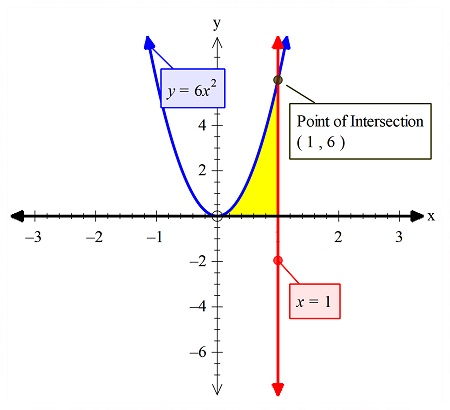# Find the volume of the solid obtained by rotating the region bounded by the curves y = 6x^2,...

## Question:

Find the volume of the solid obtained by rotating the region bounded by the curves {eq}y = 6x^2, x=1, y =0 {/eq} about the x-axis.

## Volumes of Revolution With Integration:

Out of the two methods to find the volume, we have used the disk method in the solution. The shell could have been used if the axis of rotation is parallel to the vertical axis. But since the axis of rotation is parallel to the horizontal axis, so we used the disk method.

## Answer and Explanation:The yellow region is the bounded region. The disk method is used to find the volume of revolution of this bounded region.

The outer...

See full answer below.

Become a Study.com member to unlock this answer! Create your account

#### Learn more about this topic:

How to Find Volumes of Revolution With Integration

from Math 104: Calculus

Chapter 14 / Lesson 5
11K